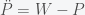# The Dynamics of Inflation

Posted on the 18 May 2022 by Ccc1685 @ccc1685

Inflation, the steady increase of prices and wages, is a nice example of what is called a marginal mode, line attractor, or invariant manifold in dynamical systems. What this means is that the dynamical system governing wages and prices has an equilibrium that is not a single point but rather a line or curve in price and wage space. This is easy to see because if we suddenly one day decided that all prices and wages were to be denominated in some other currency, say Scooby Snacks, nothing should change in the economy. Instead of making 15 dollars an hour, you now make 100 Scooby Snacks an hour and a Starbucks Frappuccino will now cost 25 Scooby Snacks, etc. As long as wages and prices are in balance, it does not matter what they are denominated in. That is why the negative effects of inflation are more subtle than simply having everything cost more. In a true inflationary state your inputs should always balance your outputs but at an ever increasing price. Inflation is bad because it changes how you think about the future and that adjustments to the economy always take time and have costs.

This is why our current situation of price increases does not yet constitute inflation. We are currently experiencing a supply shock that has made goods scarce and thus prices have increased to compensate. Inflation will only take place when businesses start to increase prices and wages in anticipation of future increases. We can show this in a very simple mathematical model. Let P represent some average of all prices and W represent average wages (actually they will represent the logarithm of both quantities but that will not matter for the argument). So in equilibrium P = W. Now suppose there is some supply shock and prices now increase. In order to get back into equilibrium wages should increase so we can write this aswhere the dot indicates the first derivative (i.e. rate of change of W is positive if P is greater than W). Similarly, if wages are higher than prices, prices should increase and we haveNow notice that the equilibrium (where there is no change in W or P) is given by W=P but given that there is only one equation and two unknowns, there is no unique solution. W and P can have any value as long as they are the same. W - P = 0 describes a line in W-P space and thus it is called a line attractor. (Mathematicians would call this an invariant manifold because a manifold is a smooth surface and the rate of change does not change (i.e. is invariant) on this surface. Physicists would call this a marginal mode because if you were to solve the eigenvalue equation governing this system, it would have a zero eigenvalue, which means that its eigenvector (called a mode) is on the margin between stable and unstable.) Now if you add the two equations together you getwhich implies that the rate of change of the sum of P and W, which I call S, is zero. i.e. there is no inflation. Thus if prices and wages respond immediately to changes then there can be no inflation (in this simple model). Now suppose we have insteadThe second derivative of W and P respond to differences. This is like having a delay or some momentum. Instead of the rate of S responding to price wage differences, the rate of the momentum of S reacts. Now when we add the two equations together we getIf we integrate this we now getwhere C is some nonnegative constant. So in this situation, the rate of change of S is positive and thus S will just keep on increasing forever. Now what is C? Well it is the anticipatory increases in S. If you were lucky enough that C was zero (i.e. no anticipation) then there would be no inflation. Remember that W and P are logarithms so C is the rate of inflation. Interestingly, the way to combat inflation in this simple toy model is to add a first derivative term. This changes the equation towhich is analogous to adding friction to a mechanical system (used differently to what an economist would call friction). The first derivative counters the anticipatory effect of the second derivative. The solution to this equation will return to a state zero inflation (exercise to the reader).

Now of course this model is too simple to actually describe the real economy but I think it gives an intuition to what inflation is and is not.

2022-05-18: Typos corrected.

See more